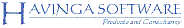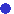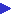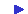Havinga SoftwareThreeDimSimFeaturesExamples...Car break thruHourglassSimple carJumping BipedMotor controlMini BipedTippe-topEnv. mappingBilliard playSatellite orbitingScreenshotHow it worksSystem modelingQuick tutorialDownloadSupport

# ThreeDimSim: Motor control

#### Introduction

In order to test the capabilities of ThreeDimSim, I studied the simulation of a digitally controlled servo motor and compared the simulation results with data obtained from an arrangement shown in figure 1. This example shows how mechanical simulation can be mixed with a simulation of a digital servo-controller.Figure 1: The servo under test.

The servo motor (with a gearbox 1:250) is driving a wooden slat of 20 cm length and 20 grams weight. The servo is controlled by a digital servo controller built with the PIC16F872.  The aim of this study is to verify the simulation and to estimate motor parameters, not to optimize the controller. For this test, I've chosen a simple behavior of the servo to perform: the slat should be turned from the left to the right side (turn from +45 degrees to -45 degrees).

#### Servo controller

The servo controller uses a straight forward (naive) control program that is given in Figure 2. Its output is proportional to the error signal that is defined as the difference between target angular position and actual position. Since available power is limited, the output is truncated to a minimum and a maximum value, as  the graph of the transfer function shows in figure 3.

```// Servo_Power is output pulse width: 0..255 = 0%..100%
Servo_Power = 255;
if (Servo_CurrentPosition < Servo_TargetPosition)
{
Servo_Cmd = 1; // set direction
// error signal:
BYTE e = Servo_TargetPosition - Servo_CurrentPosition;
if (e < 16) // set power proportional to error signal
Servo_Power = e<<4;
}
else if (Servo_CurrentPosition > Servo_TargetPosition)
{
Servo_Cmd = 2; // set direction
// error signal:
BYTE e = Servo_CurrentPosition - Servo_TargetPosition;
if (e < 16) // set power proportional to error signal
Servo_Power = e<<4;
}
else
{// correct position
Servo_Cmd = 0; // motor off
Servo_Power = 0; // no power
}
```

Figure 2: The naive servo control code implemented in the PIC 16F872Figure 3: Control function of the servo controller.

#### Measurement

The turn of the slat was registered by a video camera running at 30 fps, and the video images were used to obtain the angular data of the slat during the move. Results of this measurement is shown in figure 4. Only one turn was measured. Of course more measurements would give a smaller error, but due to lack of time I left it by just one run.  Note the system shows a big overshoot and some oscillation.Figure 4: Measured response of of the servo system.

#### Simulation

The simulation was performed with ThreeDimSim version 1.0.9.0 with the script servo_estimate.thd. The script describes a geared dc motor by assigning a typical torque-function to the torque member of a joint. The torque-function is defined as Tm=Vin-Kb*w*N*(Km/Ra). This function is derived from a general well known dc-motor model shown in figure 5. In this function I also account for the gear-ration 1:N. Furthermore, the motor inertia is modeled by an extra cylindrical load, with a diameter that has to be estimated from the data.  The motor control function is copied form the actual motor control program running on the PIC16F872. The controller output is a pulse-width modulated current, and I model that by switching the torque on and off. In this case no electrical motor breaking is used.Figure 5: Model of the dc-motor used. Note the script describes torque Tm as function of Vin and the speed of the shaft. The motor inertia Jm and load inertia, as well as friction fm is modeled separately.

The script measures the difference between simulated output and measured output in terms of a sum of squares of differences. This fit is used to estimate the parameters Kb(=Km), Ra, motor friction factor and motor inertia by means of the 'solve' function. This is done at increasingly small integration step sizes.

#### Simulation results

The motor parameters estimated are given in the table below. These values were obtained with a simulation step size of 1e-4 seconds.

 Parameter Estimated value Kb 3.1e-3 Ra 72e-3 Jm 1.0e-6 fm 52e-6

The fit achieved is  ssd=46.25, and corresponds to a std error of 1.25 degrees.  The response curves (figure 7) show a reasonably good fit with the real-world data. Figure 6 shows the simulation result with the original measurement video as background. (This video background is in fact a video texture in the 3D-scene).Figure 6: Simulated response displayed with video as backgroundFigure 7:  The measured (blue) and simulated (red) response of the servo system

The error in simulation could be explained by different sources. The model could be not adequate enough to obtain a better fit, but also it seems that the integration with smaller step sizes give slightly better results. Furthermore the data is taken from one run only. It more runs were taken into account, the measurement error would be less, and this could make it possible to obtain a better fit.